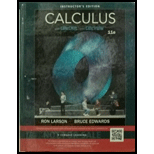To prove: The equation ∫ a b y d x = ∫ t 1 t 2 g ( t ) f ′ ( t ) d t when y = g ( t ) and x = f ( t ) , a ≤ x ≤ b and f ( t 1 ) = a , f ( t 2 ) = b both the functions g and f ′ are continuous on [ t 1 , t 2 ] .Calculus (MindTap Course List)

11th Edition
Ron Larson + 1 other
Publisher: Cengage Learning
ISBN: 9781337275347Calculus (MindTap Course List)

11th Edition
Ron Larson + 1 other
Publisher: Cengage Learning
ISBN: 9781337275347

Solutions

Chapter 10.3, Problem 77E
To determine

Expert Solution

Want to see the full answer?

Check out a sample textbook solution.

Want to see this answer and more?

Experts are waiting 24/7 to provide step-by-step solutions in as fast as 30 minutes!*

*Response times may vary by subject and question complexity. Median response time is 34 minutes for paid subscribers and may be longer for promotional offers.